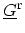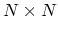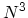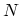## 4.7.3 Discussion

The carrier concentration is related to the diagonal elements of the GREEN's function. The calculation of the current requires only the nearest off-diagonal elements of the GREEN's function. Furthermore, the HAMILTONian matrix is tridiagonal. Considering these factors one can employ an efficient method, such as the recursive GREEN's method, to calculate only the required elements of the GREEN's functions.

The recursive method has been proposed in [116,8]. A brief description of the method is presented in Appendix H. The operations required to solve for all elements ofwith a size ofscales as. However, the required operations for the recursive method scales linearly with.

M. Pourfath: Numerical Study of Quantum Transport in Carbon Nanotube-Based Transistors